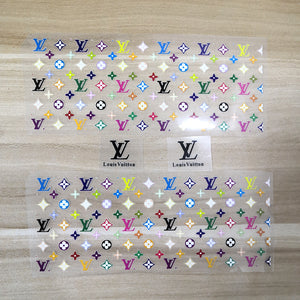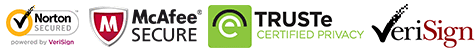free shipping this Week On order over USD120
free shipping this Week On order over USD120# Colorful LV Patches for Custom Air Force 1 LV, Rainbow LV Patches For Shoes Decal

Regular price \$15.00 \$9.90 Save \$5.10

ONLY 4598915 LEFT

 Hours   Minutes   Seconds

Sale Ends Once The Timer Hits Zero!

ONLY 4598915 LEFT

Get these easy use iron on coloful Louis Vuitton patches to create your own colorful LV shoes. Best size for DIY or custom  Air Force 1 & Vans.

<<<<<<<<<<<<<<<<<<<<<<<<<<<<<<<<<<<<<<<<<<<<<<<<<<<<<<<

Please STOP wasting money to buy the "NO RETURN, NO REFUND colorful lv shoes. Try this iron on lv stickers to DIY. Save lots of money!

<<<<<<<<<<<<<<<<<<<<<<<<<<<<<<<<<<<<<<<<<<<<<<<<<<<<<<<

Why use these colorful LV patches:

• 1. Perfect sizes for sneakers and other items, such as socks, hoodies, jeans, jackets....
• 2. Waterproof and flexible, when iron on, proved durable after washing test and brushing test. You can check the videos at the bottom of front page or my YouTube Channel: www.youtube.com/c/theshoesgirl
• 3. Easy use, just iron on directly, no need any transfer paper, no need any sealer. Will not peel off even brushing in water! You can watch the test in my videos.
• 4. Durable but still can be removed, if you are tired with this design after several months, remove them and change another design to refresh your shoes.

<<<<<<<<<<<<<<<<<<<<<<<<<<<<<<<<<<<<<<<<<<<<<<<<<<<<<<<

• ### The set includes:

• 2 sheets of colorful LV patches
• 2 black " Louis Vuitton"

Also have another design with white background:

https://www.theshoesgirl.com/products/colorful-lv-patches-with-white-background

<<<<<<<<<<<<<<<<<<<<<<<<<<<<<<<<<<<<<<<<<<<<<<<<<<<<<<<
• ### Size:

#### LV sheet:

width 18 cm; height: 7 cm

<<<<<<<<<<<<<<<<<<<<<<<<<<<<<<<<<<<<<<<<<<<<<<<<<<<<<<<

### How to use:

1. Position the lv patches on the shoes with tape, mark on the tape, then cut along with the marks.

2. Iron on the rest part of the patches, please keep temperature 135-150℃

3. peel the vinyl, the rest of lv patches will stick on the shoes.

4. If use normal iron, please turn off the steam, keep the temperature above, use the tip and be careful and patient, for it's heavy to control, better use the mini iron.

5. Watch the tutorial video here: